# Triangles

Go back to  'Geometry'

 1 Introduction to Triangle 2 What is a Triangle? 3 Different Types of Triangles 4 Fun Facts on Triangles 5 Properties of a Triangle 6 Formulas of a Triangle 7 Tips and Tricks 8 Solved Examples on Triangles 9 Important Notes on Triangles 10 Practice Questions on Triangles 11 Challenging Questions on Triangles 12 Important Topics of Triangles 13 Maths Olympiad Sample Papers 14 Frequently Asked Questions (FAQs) 15 Download FREE Worksheets of Triangles

We at Cuemath believe that Math is a life skill. Our Math Experts focus on the “Why” behind the “What.” Students can explore from a huge range of interactive worksheets, visuals, simulations, practice tests, and more to understand a concept in depth.

Book a FREE trial class today! and experience Cuemath’s LIVE Online Class with your child.

## Introduction to Triangle

A closed figure made with 3 line segments forms the shape of a triangle.

Triangles are an important part of our lives.

You can observe the shape of a triangle in signboards, your favourite sandwiches or birthday banners.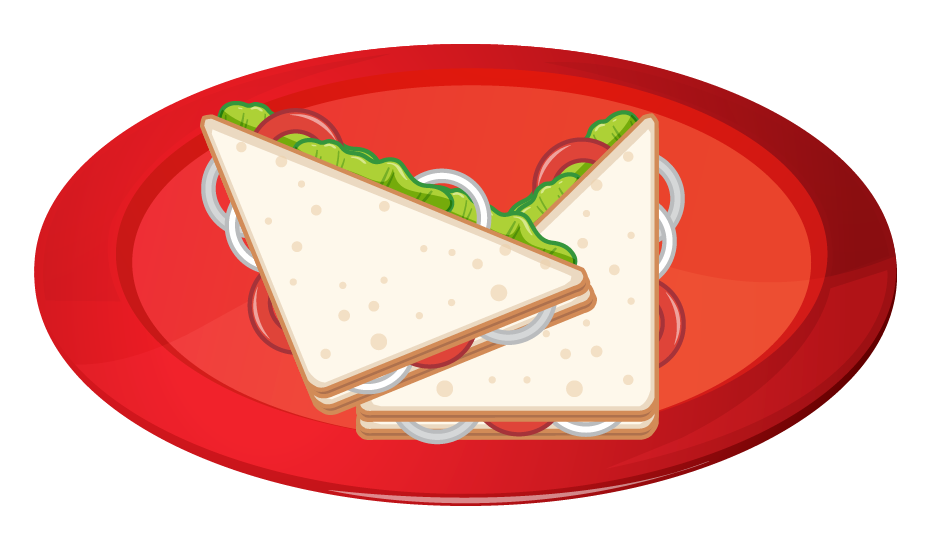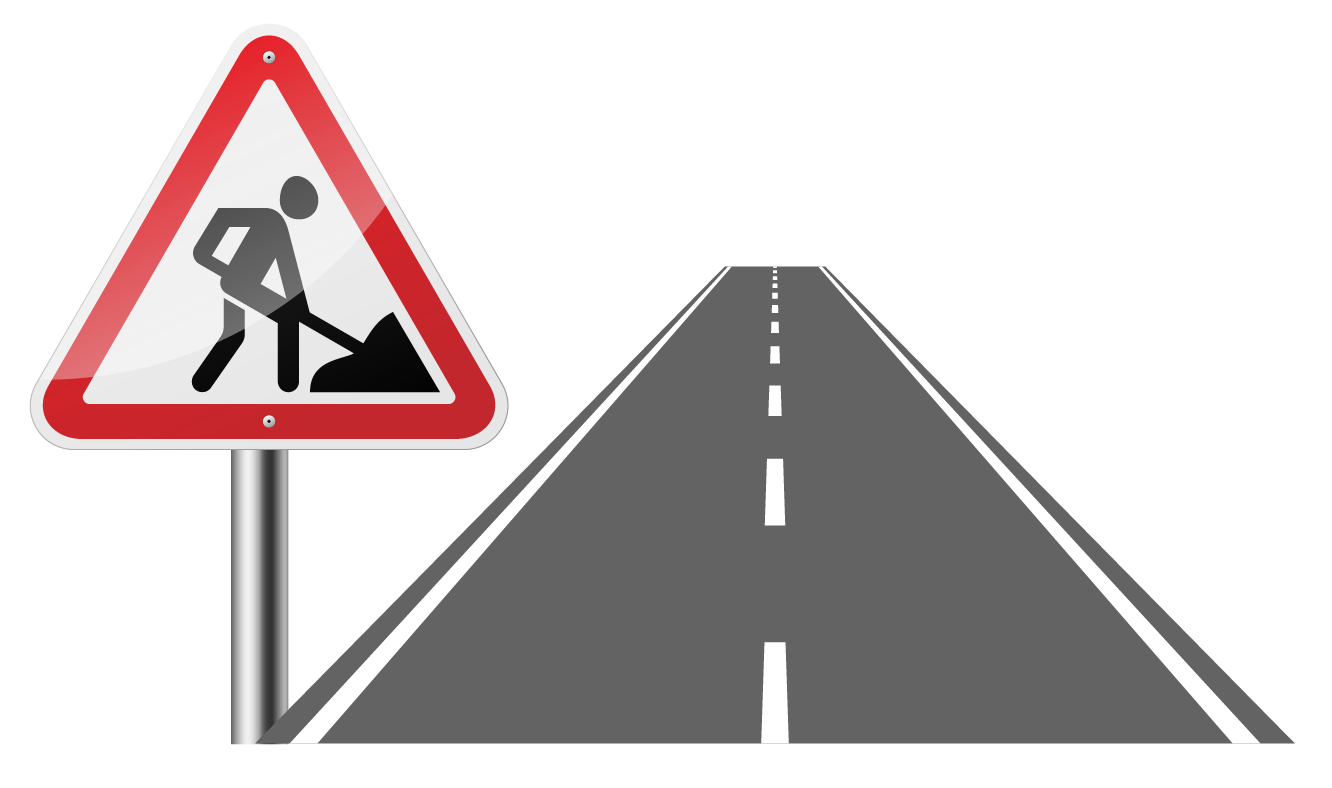## What is a Triangle?

A triangle is a closed figure or shape with 3 sides, 3 angles and 3 vertices.

A triangle with vertices P, Q, and R is denoted as △PQR.

The elements of a triangle are its 3 angles and its 3 sides.

Let's look at triangle PQR.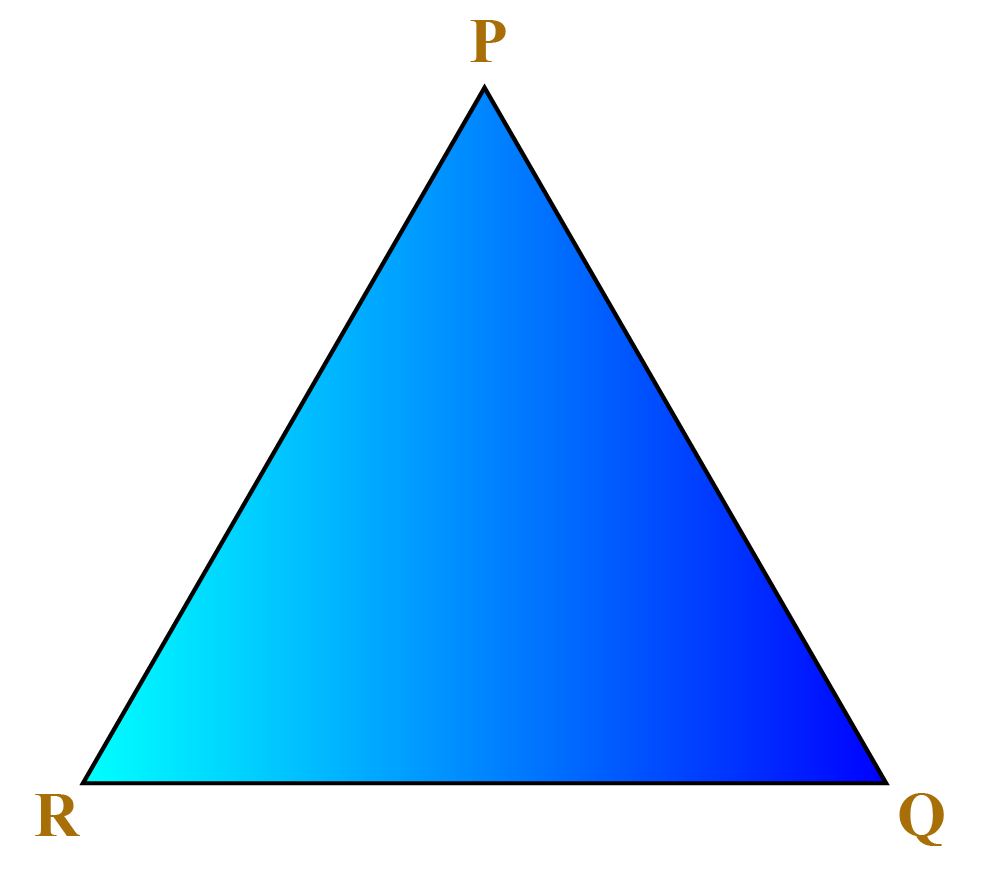The elements of this triangle are:

• $$\angle PQR$$
• $$\angle QRP$$
• $$\angle RPQ$$
• PQ
• QR
• RP

You can explore the relation between the elements of a triangle by dragging the vertices of the triangle shown below.

## Different Types of Triangles

Triangles can be classified based on their angles or the length of their sides.

### Classification of Triangles by Angles

Type of Triangle  Example Explanation
Right-angled triangle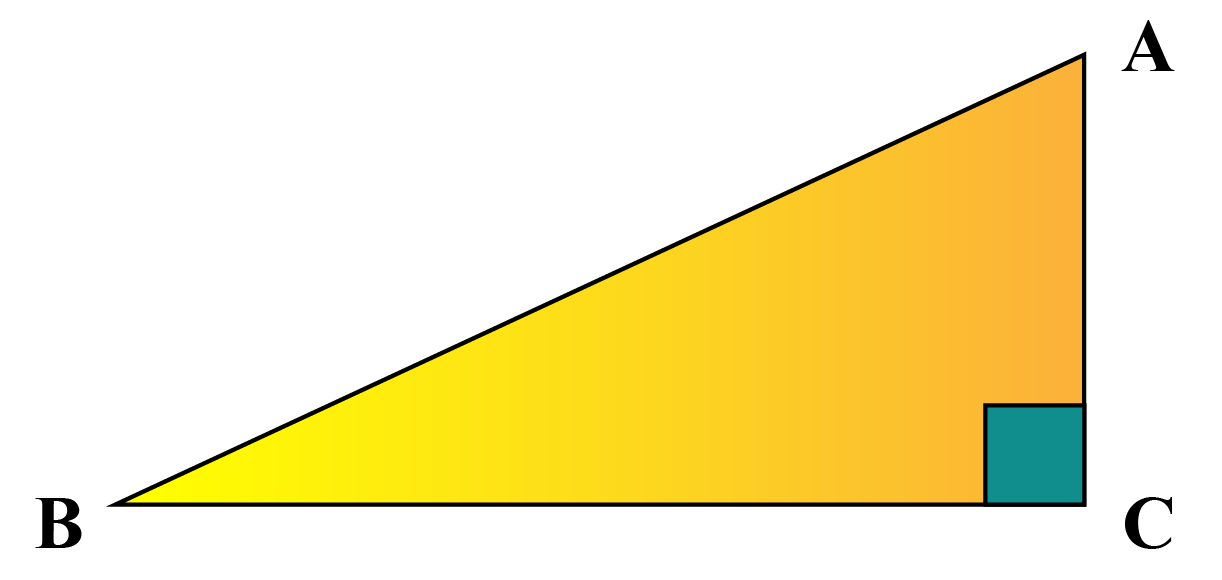A right triangle has one of its vertex angles as 90°.

In the above triangle, $$\angle \text{ACB}$$ = 90°.

Acute-angled triangle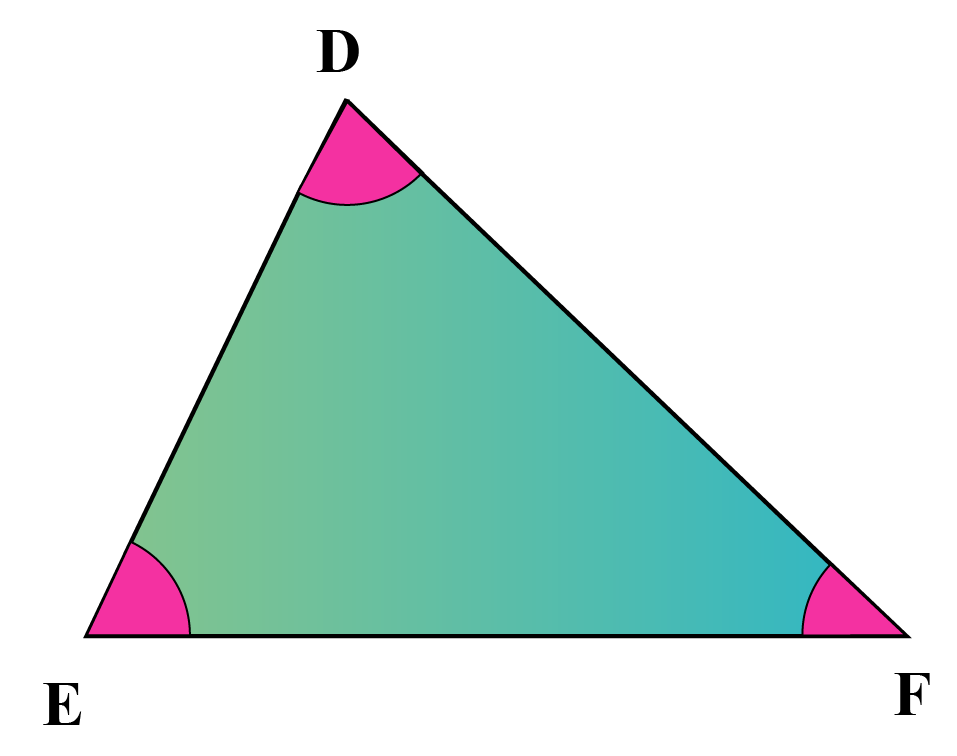An acute triangle has all its internal angles as acute( i.e.. less than 90°).

Obtuse-angled triangle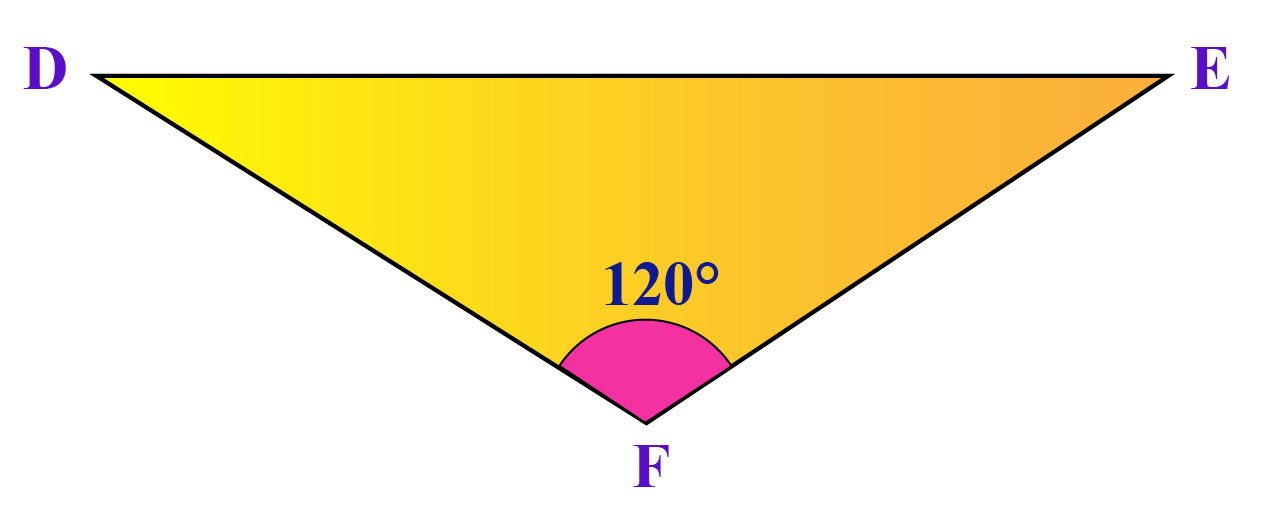An obtuse triangle has one of its internal angles as obtuse ( i.e.. greater than 90°).

In the given triangle, $$\angle \text{DFE}$$= 120$$^\circ$$

### Classification of Triangles by Sides

Type of Triangle  Example Explanation
Equilateral triangle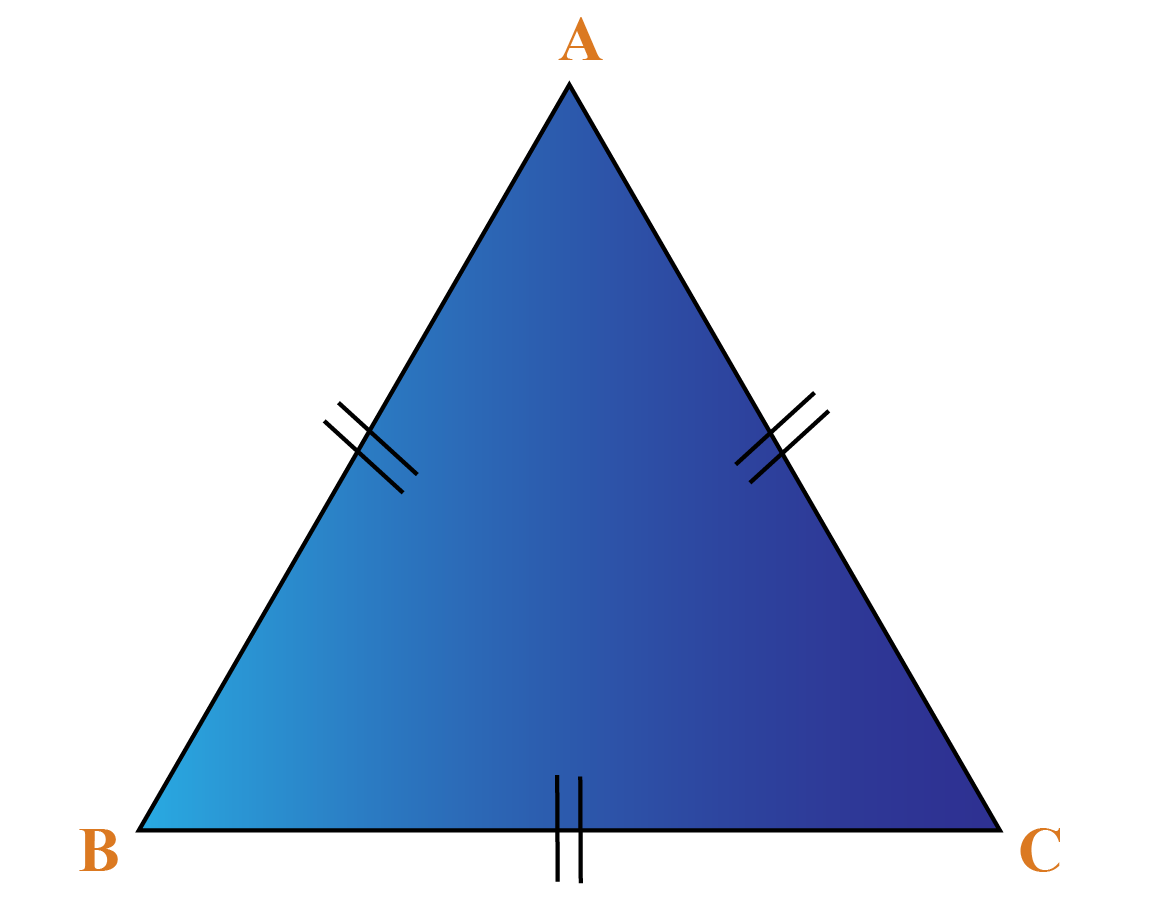If all the three sides of a triangle are equal, it is called an equilateral triangle.

In the given triangle, all three sides are equal in length.

AB = BC = AC

Isosceles triangle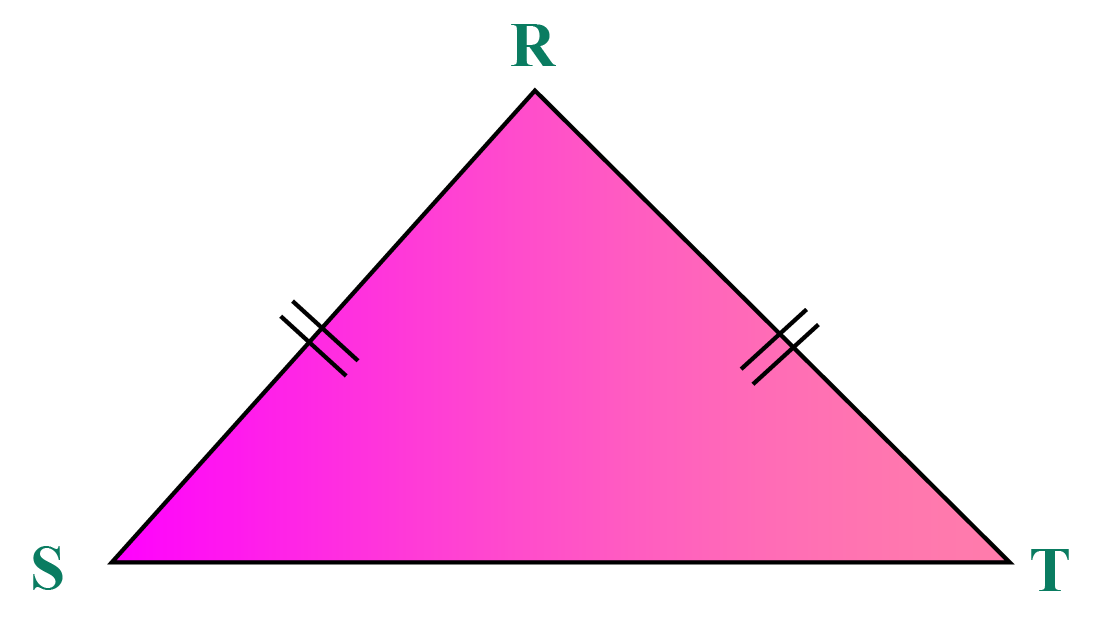If any two sides of a triangle are equal, it is called an isosceles triangle.

In the given triangle, two sides are equal.

RS = RT

Scalene triangle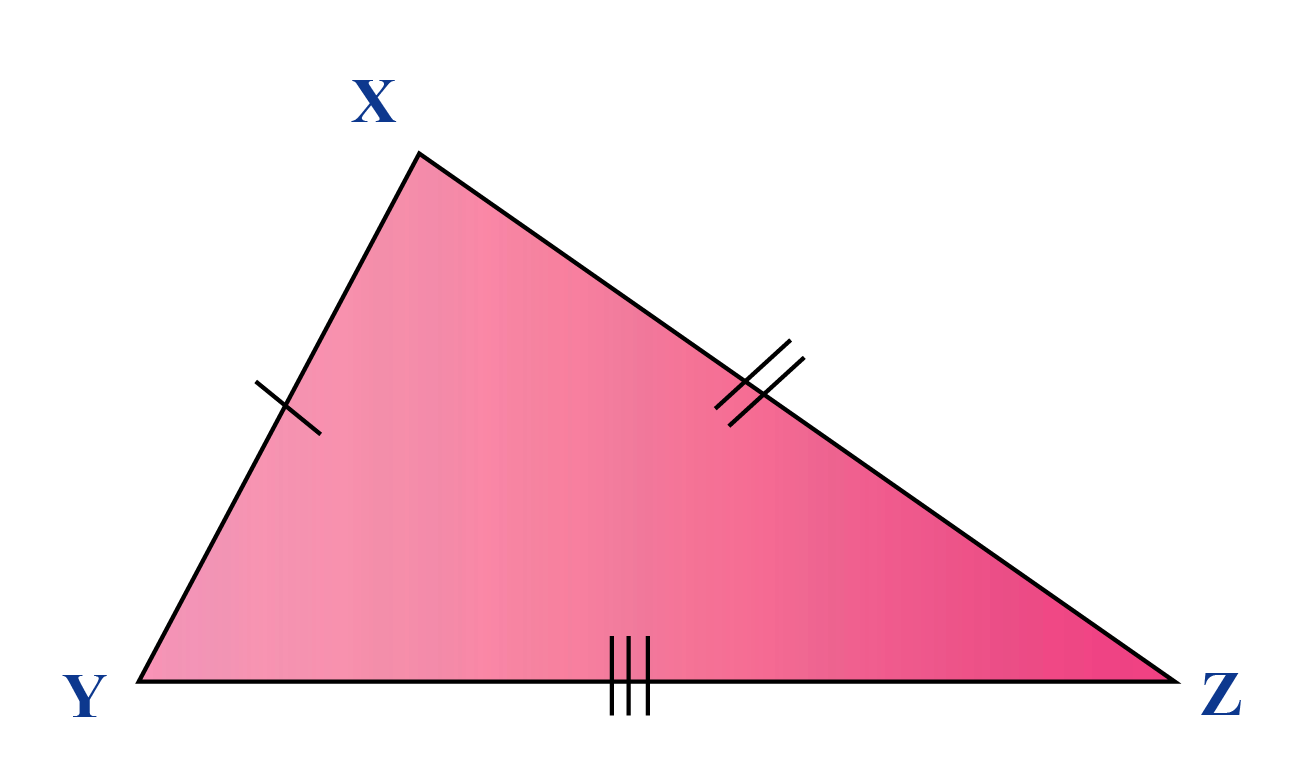If a triangle has three unequal sides, it is called a scalene triangle.

In the given triangle, all three sides are of unequal lengths.

XZ ≠ XZ ≠ YZ

## Fun Facts on Triangles

• A triangle can always be divided into two right triangles, irrespective of its orientation.
• Triangles are polygons with the least number of sides. There are no polygons with less than 3 sides.
• Many towers, buildings and monuments are constructed in the shape of triangles to give them more stability and strength.
Here are few examples for you:1. Eiffel Tower

The world-famous Eiffel Tower is triangular in shape and has around 186 triangles in its structure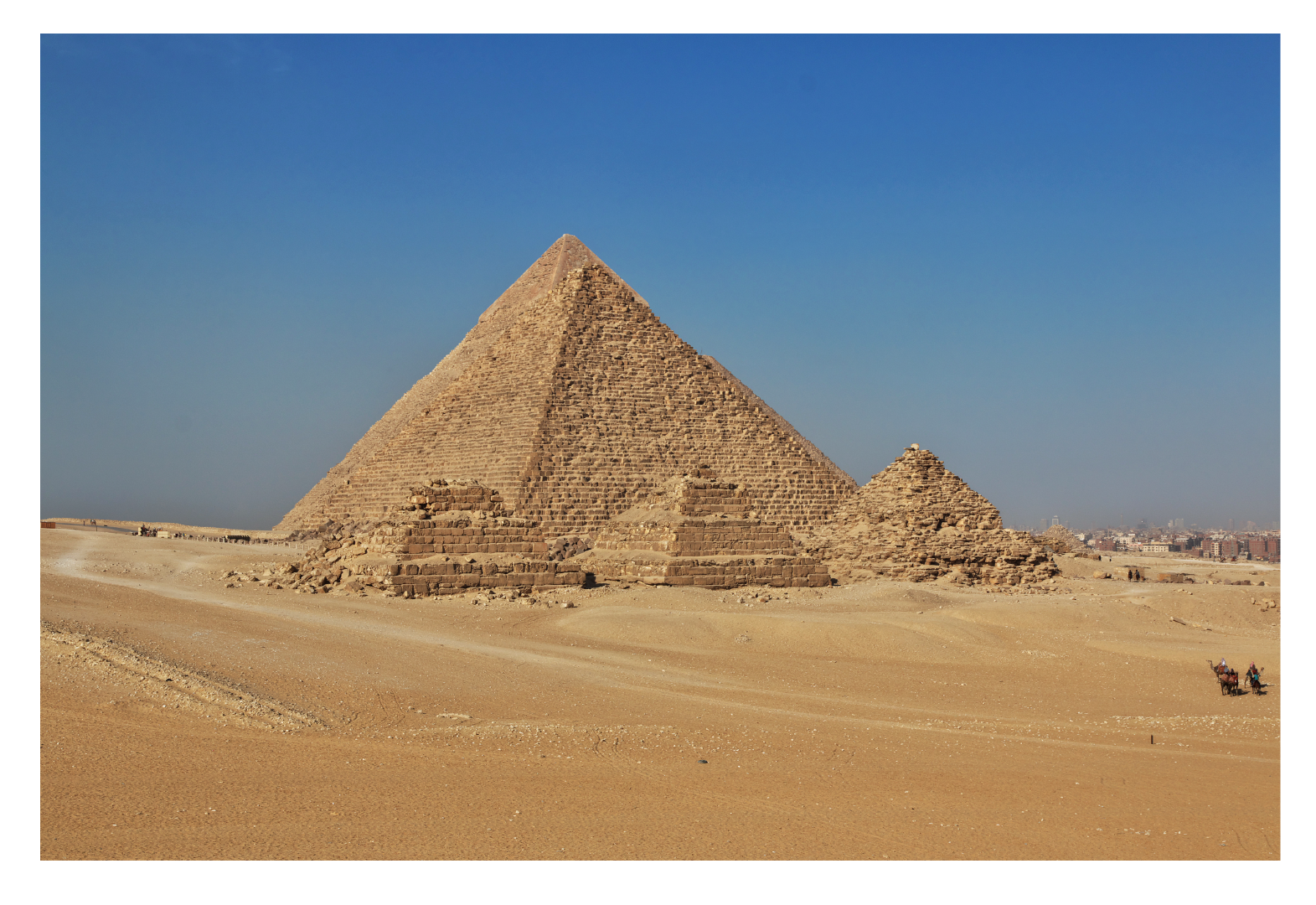2. Egyptian Pyramids

They have triangular faces3. Bridges

They use triangular frames for stability

## Properties of a Triangle

Some of the important properties of a triangle are listed below.

### Property 1 - Angle Sum Property

The Angle Sum Property states that the sum of the three interior angles of a triangle is 180°In the given triangle,

$$\angle \text{D} + \angle \text{E} + \angle \text{F} = 180^\circ$$

### Property 2 - Triangle Inequality Property

The Triangle Inequality Property sum of the length of the two sides of a triangle is greater than the third side.in $$\Delta PQR$$

• p + q > r  ( 6 + 4 > 3)
• r + p > q (3 + 4  > 6)
• r + q > p ( 6 + 3 > 4)

### Property 3 - Pythagorean Theorem

The Pythagorean theorem states that in a right triangle, the square of the hypotenuse is the sum of the squares of the other two sides.

 \begin{align}\text{Hypotenuse}^2 &\!=\!\text{Base}^2 \!+\!\text{Altitude}^2 \end{align}

### Property 4 - Side opposite the greater angle is the longest side

To understand the side opposite the greater angle is the longest side property, let's consider the below-given triangle.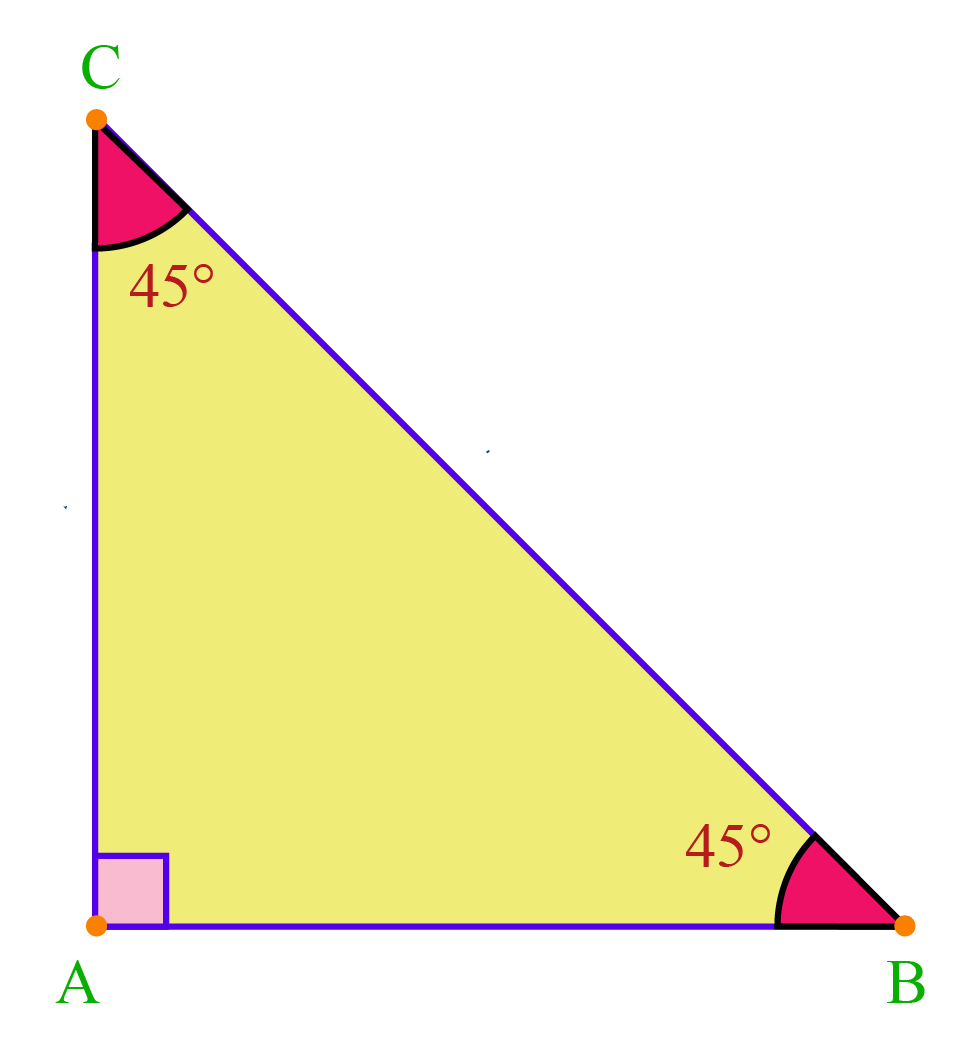In this triangle, $$\angle \text{A}$$ is the greatest angle.

Thus, side BC is the longest side.

### Property 5 - Exterior Angle Property

The Exterior Angle Property of a triangle is always equal to the sum of the interior opposite angles.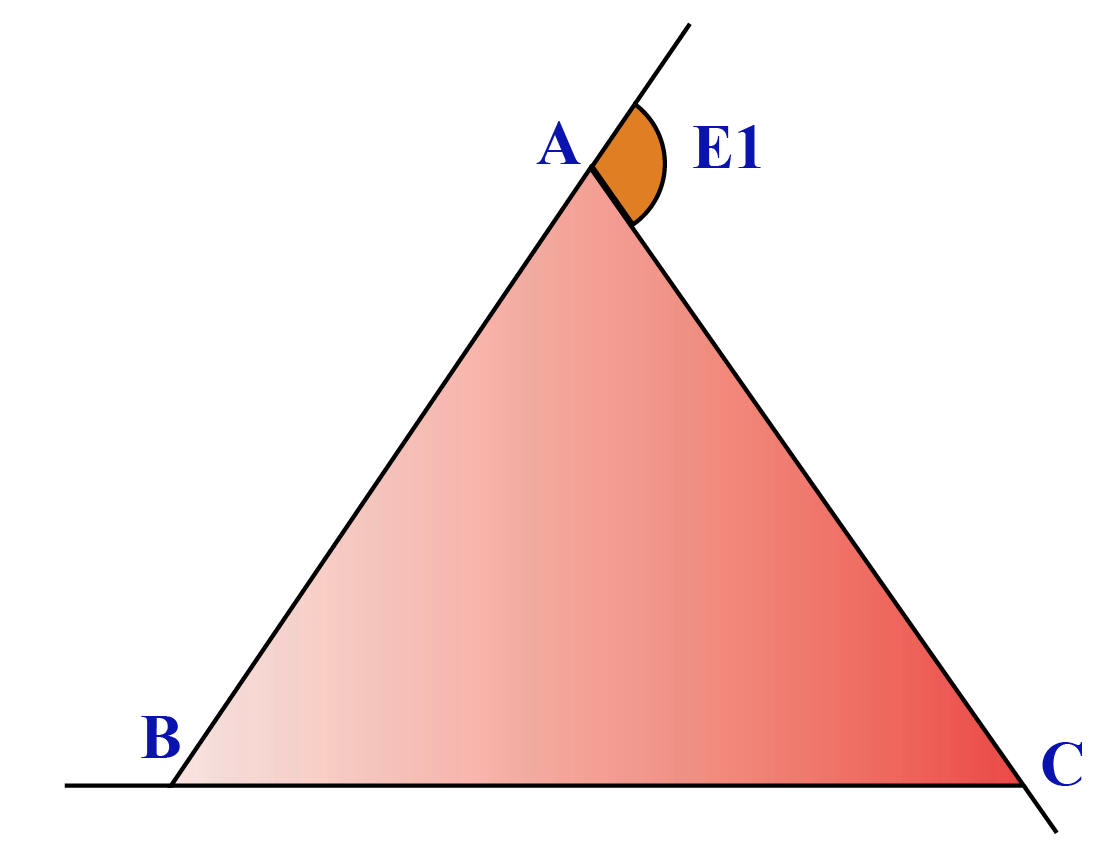In the given triangle, Exterior angle E1  = $$\angle \text{ABC} + \angle \text{BCA}$$

A triangle has 3 exterior angles and these exterior angles add up to 360° for any polygon.

### Property 6 - Congruence Property

In the Congruence Property, two triangles are said to be congruent if all their corresponding sides and angles are congruent.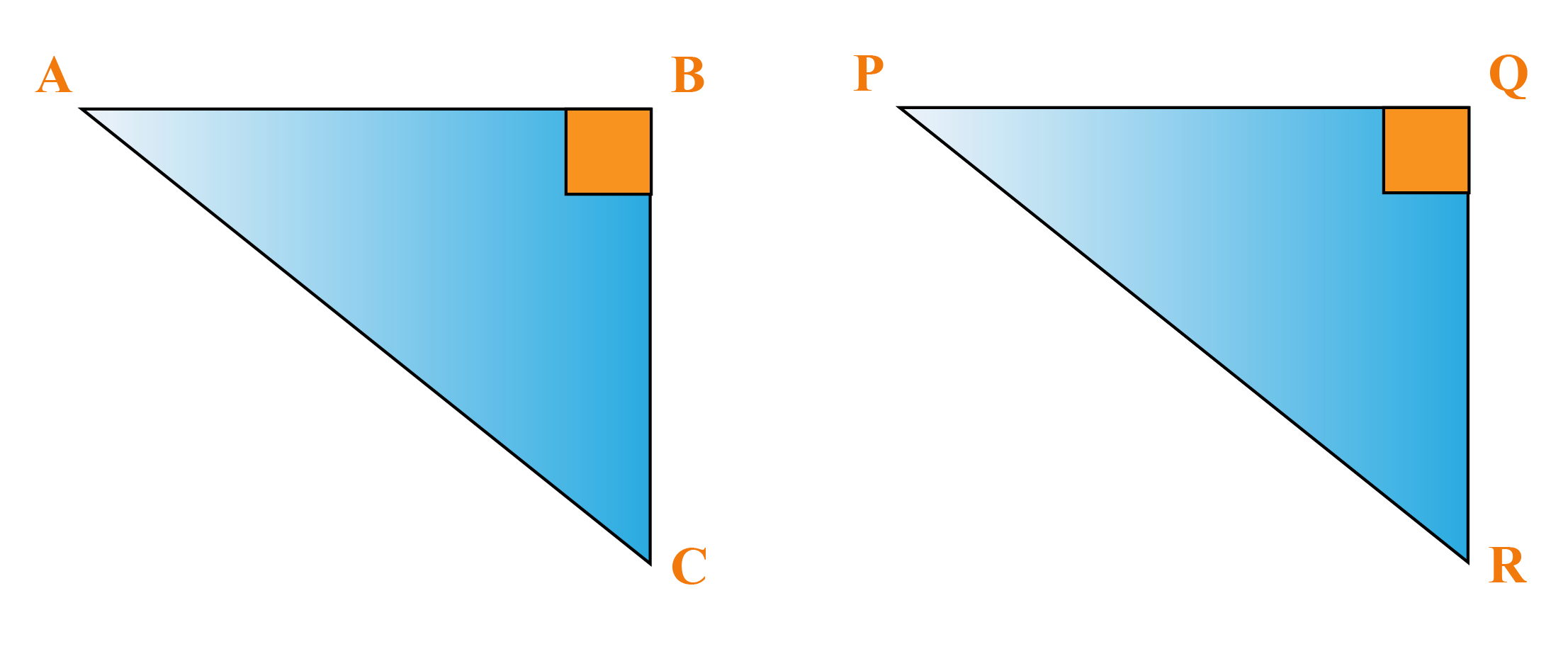All  the corresponding angles and sides are equal,

• $$\angle\text{ ABC} = \angle\text{PQR}$$
• $$\angle\text{ BAC} = \angle\text{QPR}$$
• $$\angle\text{ BCA} = \angle\text{QRP}$$
• AB = PQ
• AC = PR
• BC = QR

## Formulas of a Triangle

The formulas of a triangle help us determine its area and perimeter.

### Perimeter of a Triangle

Consider △XYZ.

The length of the sides of the triangle are represented as ‘a’, ‘b’ and ‘c’ respectively.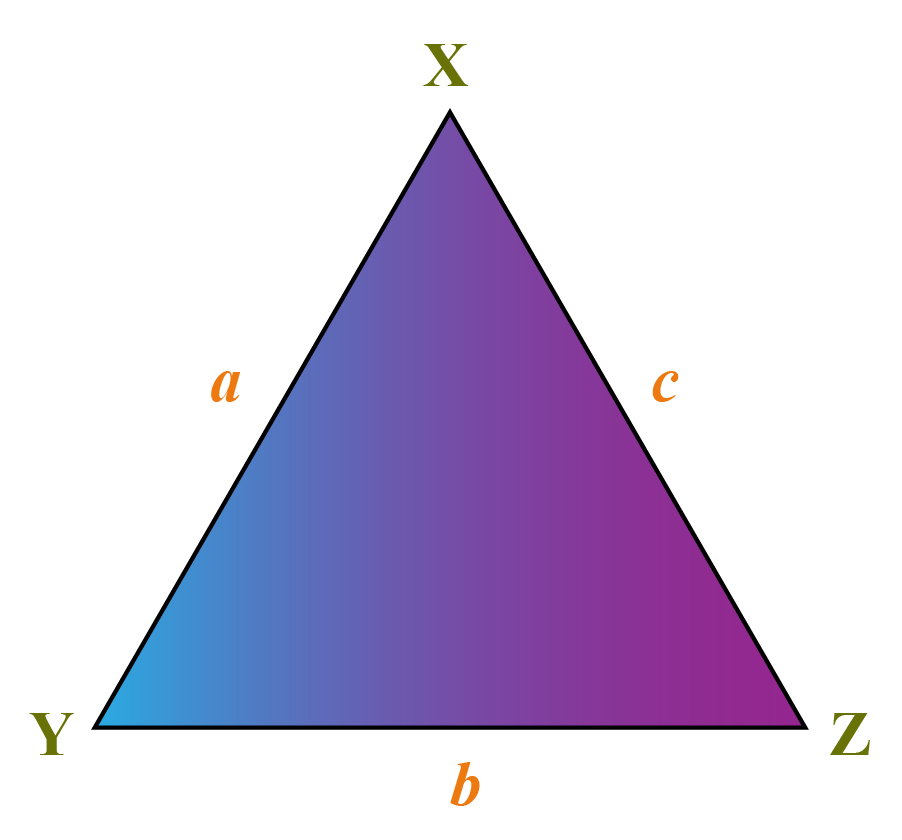$$\text{Perimeter of Triangle} = a + b + c$$

### Area of a Triangle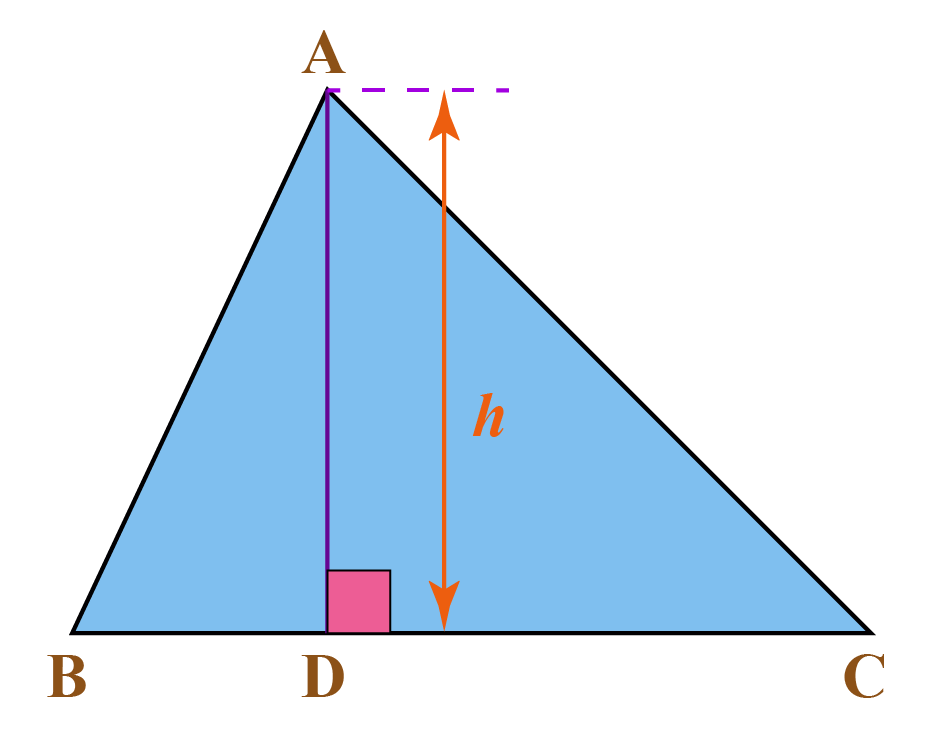In the given triangle $$ABC$$,

\begin{align}\text{Area of }\Delta ABC = \frac{1}{2} h\times \text{BC}\end{align}

$$\text{BC}$$ is the base and $$h$$ is the height of the triangle.

 \begin{align}\frac{1}{2} \times \text{Base} \times \text{Height}\end{align}Tips and Tricks
1. A triangle cannot have more than one right angle.
2. Each angle of a triangle cannot be less than 60°.
3. The angles opposite to the equal sides of an isosceles triangle are equal.

Help your child score higher with Cuemath’s proprietary FREE Diagnostic Test. Get access to detailed reports, customised learning plans and a FREE counselling session. Attempt the test now.

## Solved Examples

 Example 1

The perimeter of a triangular garden is given as 13 m.

If two of its sides measure 3 m and 4 m respectively, what is the measure of the third side?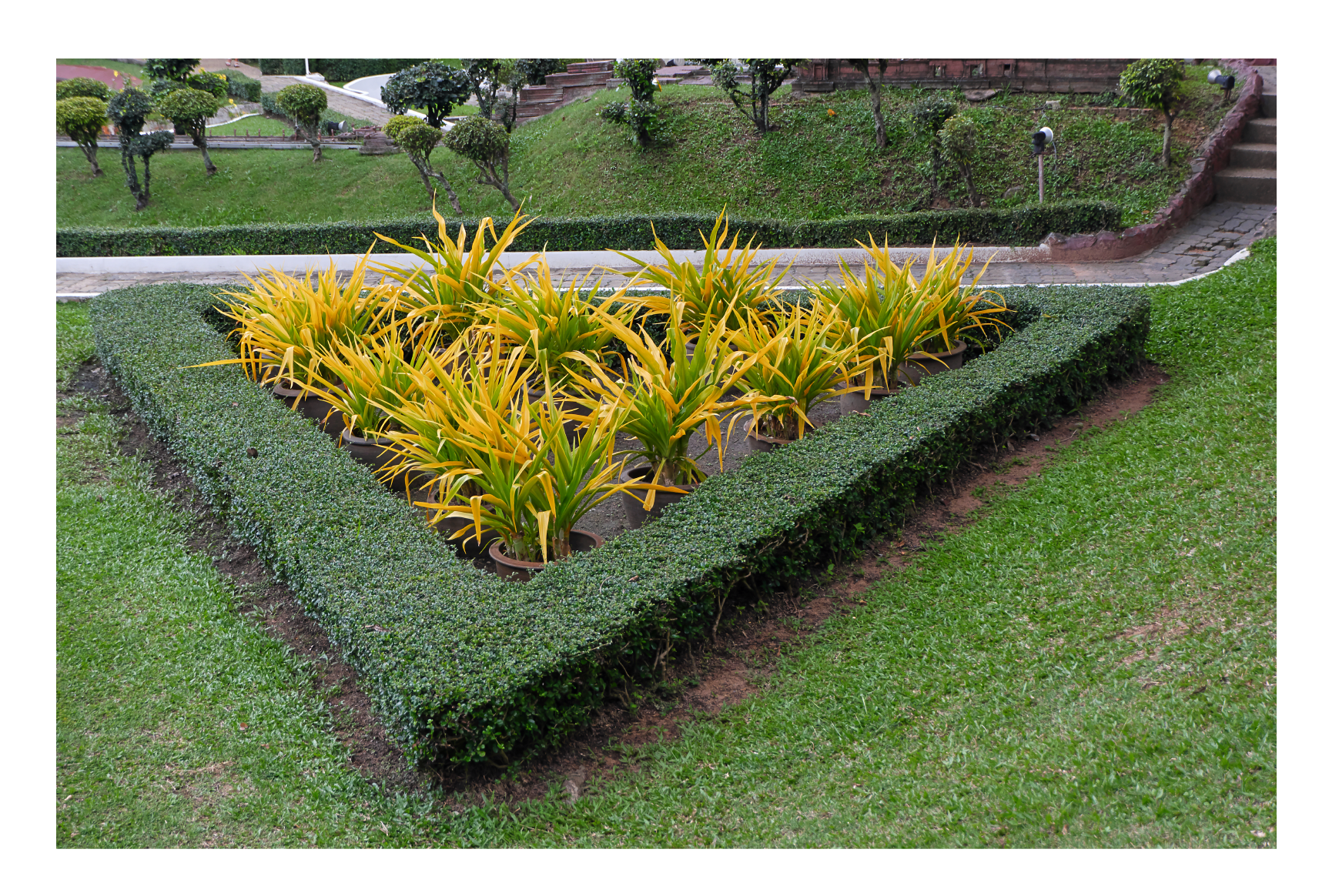Solution:

We know that the perimeter of a triangle is the sum of all three sides

$$\implies$$ 13 = 3 + 4 + unknown side

Therefore, the unknown side is given by:

\begin{align} &=13 \!-\! (3 \!+\! 4) \\ &= 6 \;m \end{align}

 $$\therefore$$The third side of the park measures 6 m
 Example 2

Tina is building a triangular, wooden birdhouse as shown.

If two of the angles measure 46° and 62°, what is the measure of the third angle?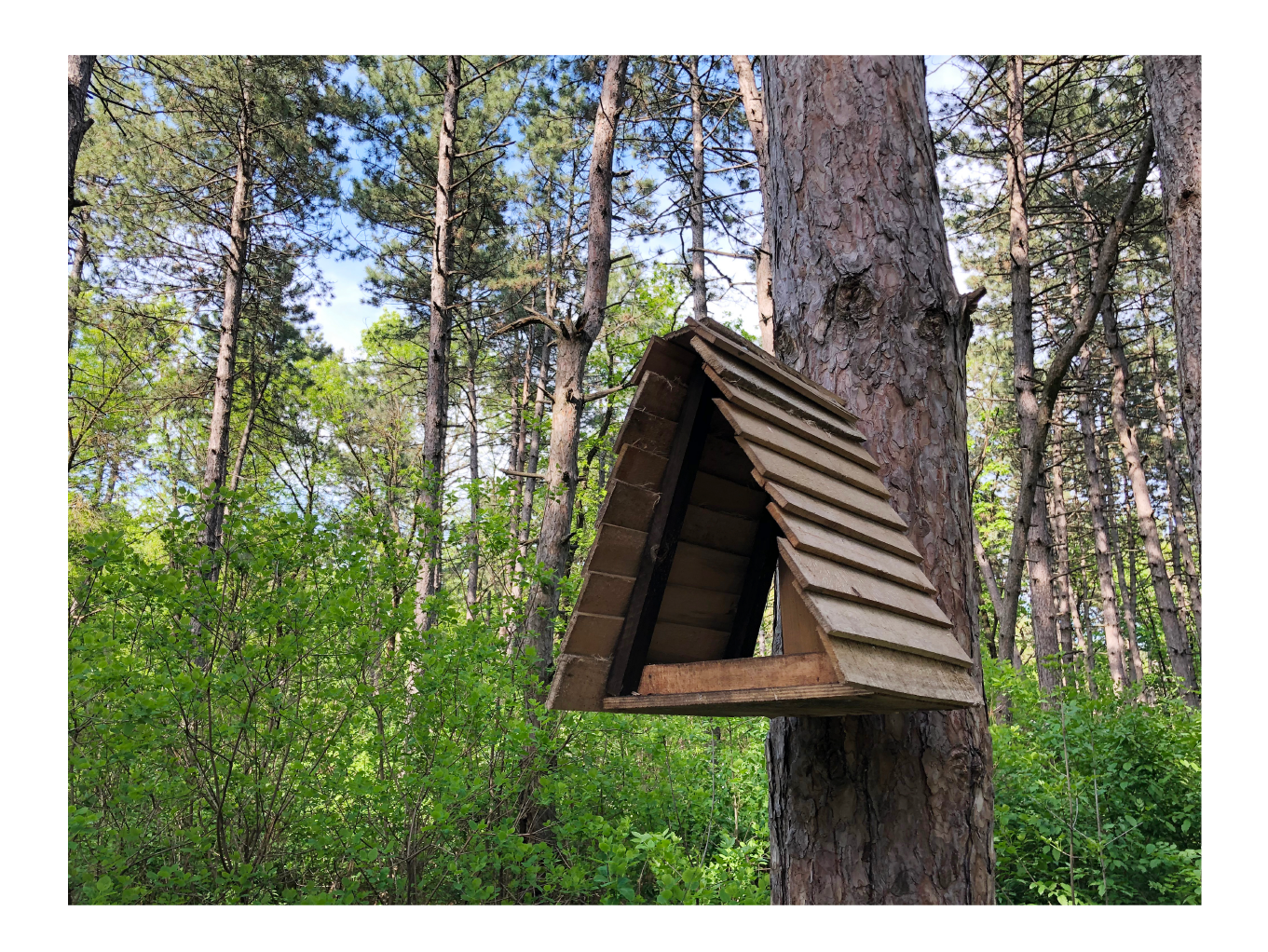Solution:

We know that the sum of the angles of a triangle add up to 180°.

Therefore, the unknown angle is:

\begin{align} &=180^\circ \!-\! (46^\circ \!+\! 62^\circ) \\ &= 72^\circ\end{align}

 $$\therefore$$The third angle measures 72°
 Example 3

In a right triangle, two of the sides measure 3 cm and 4 cm.

What is the measure of the hypotenuse?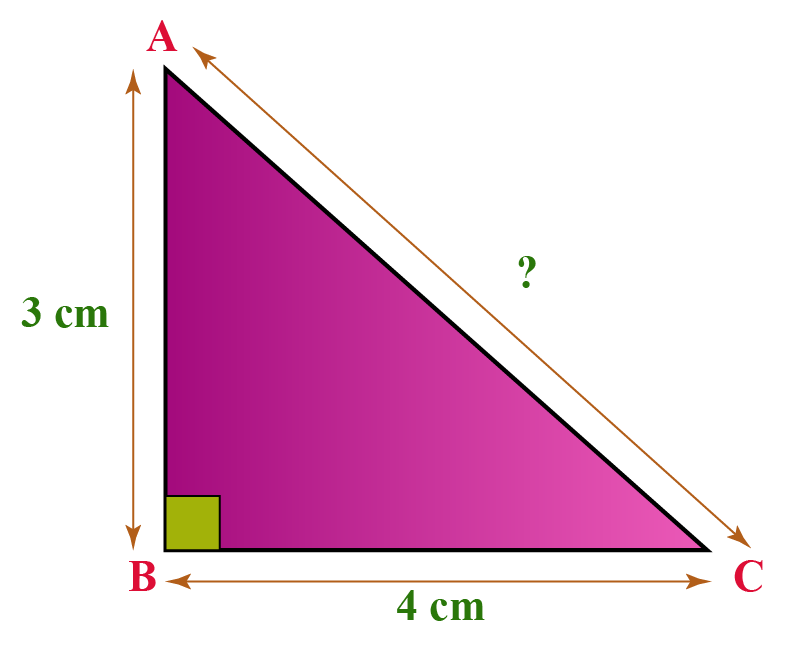Solution:

By Pythagoras theorem, we know that, hypotenuse2 = base2 + height2

Substituting the values, we get:

\begin{align} \text{hypoteneuse}^2 &=3^2 +4^2 \\ &= 9 + 16\\ &= 25\: \text{cm} \\ \implies \text{hypoteneuse} &= 5 \:\text{cm} \end{align}

 $$\therefore$$ Hypotenuse = 5 cm
 Example 4

One of the acute angles of a right-angled triangle is 45°

Find the other triangle.

Identify the type of triangle thus formed.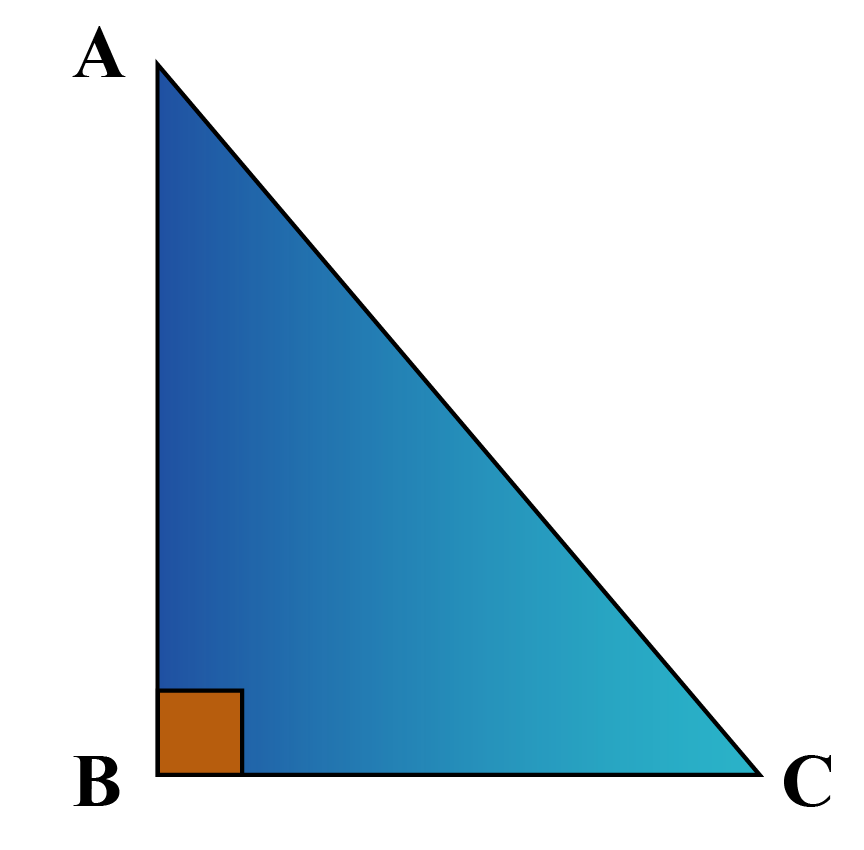Solution:

Given, angle1 =  90° and angle2 = 45°

We know that the sum of the angles of a triangle add up to 180°.

Therefore,

\begin{align} \text{angle}_3 &=180^\circ \!-\! (90^\circ \!+\! 45^\circ) \\ &= 45^\circ\end{align}

Since two angles measure the same, it is an isosceles triangle.

 $$\therefore$$ △ABC is an isosceles triangle
 Example 5

The sides of a triangular park are in the ratio 3: 4: 5 and its perimeter is 1080 m.

Find the area of the triangular park.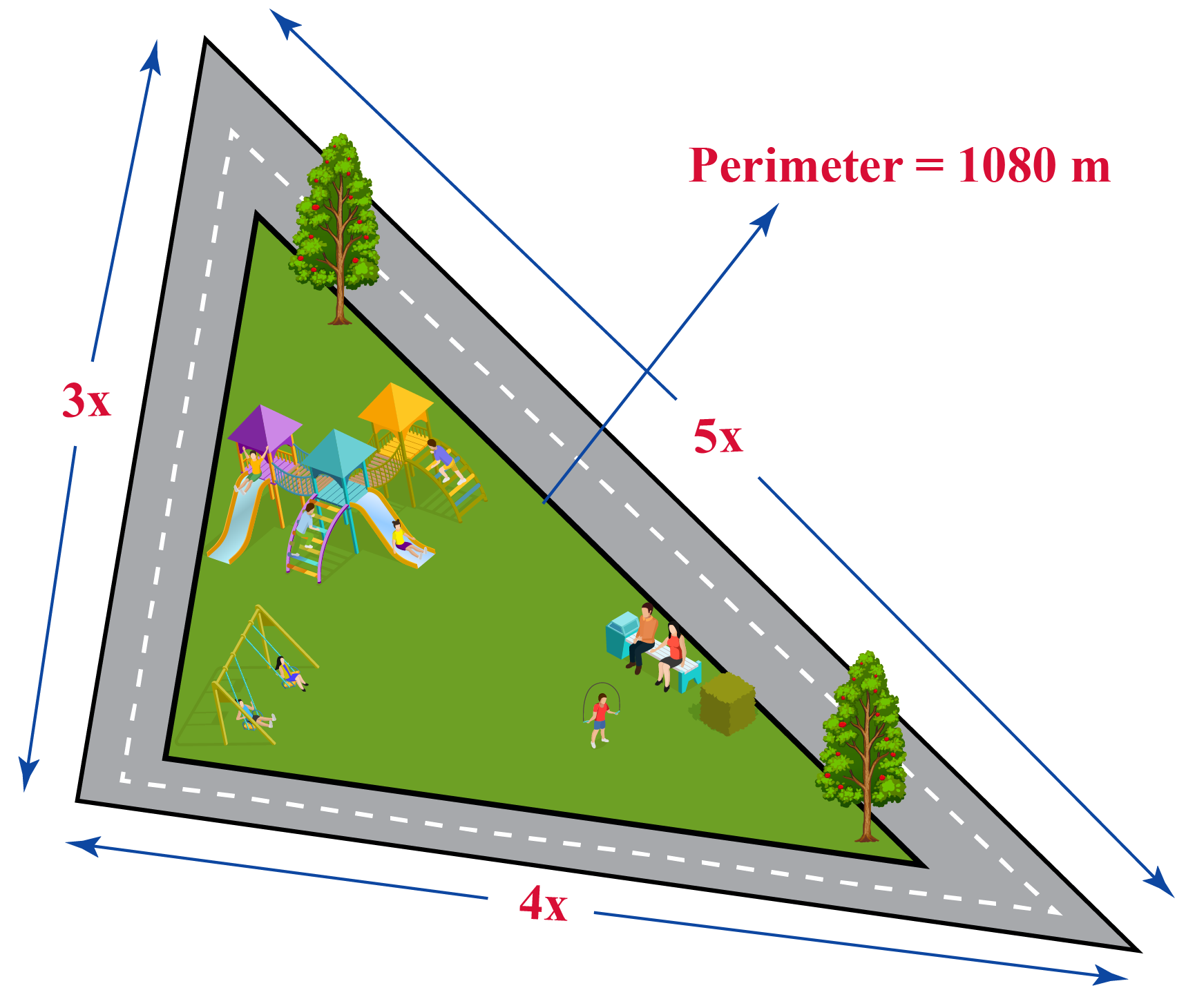Solution:

Given:

• Perimeter = 1080 m
• Sides of the triangle are in the ratio 3:4:5.

Therefore, the sides of triangle are

\begin{align} \text{side}_1 &= \frac{3}{12}\times 1080\\\ &= 270 \text{m}\\\\ \text{side}_2 &= \frac{4}{12}\times 1080\\\ &= 360\text{m} \\\\ \text{side}_3 &= \frac{5}{12}\times 1080\\\ &= 450\text{m} \end{align}

\begin{align} \text{Area of the park} &= \frac{1}{2} \times 270 \times 360 \\ &= 48600\: \text{m}^2\end{align}

 $$\therefore$$Area of the park = 48,600 m2

CLUEless in Math? Check out how CUEMATH Teachers will explain Triangles to your kid using interactive simulations & worksheets so they never have to memorise anything in Math again!

Explore Cuemath Live, Interactive & Personalised Online Classes to make your kid a Math Expert. Book a FREE trial class today!

## Practice Questions

Here are few questions for you to practice.

Area of a triangle worksheets are available at the end of the page for you to try and solve!Challenging Questions

 1. Anu said a triangle has at least one set of parallel lines. Is she right?  Why?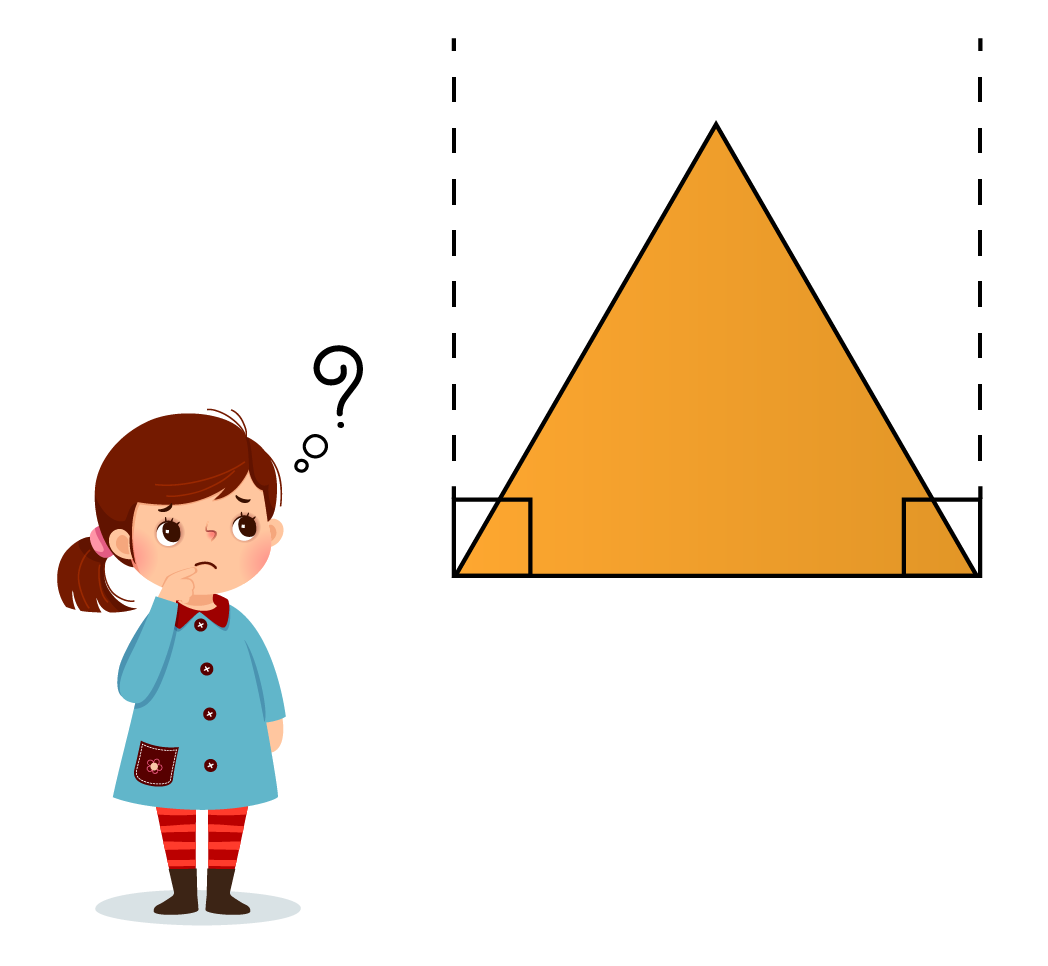2. Can a triangle be formed with dimensions 17cm, 9 cm and 30cm?

## Important Topics

Given below are the list of topics that are closely connected to triangles. These topics will also give you a glimpse of how such concepts are covered in Cuemath.

 What is Congruence? Congruence in Triangles The SAS Criterion Is There An SSA Criterion? Perpendicular Bisectors The ASA Criterion The ASA Criterion Proof Is There An AAS Criterion? Isosceles Triangles The SSS Criterion The SSS Criterion Proof The RHS Criterion The RHS Criterion Proof Relative Magnitudes of Sides and Angles The Triangle Inequality Distance of a Point From a Line Angle Bisector Triangles - Same Base-Same Parallels Pythagoras Theorem Triangle Areas - Basic Calculations Heron's Formula Basic Triangle Constructions Advanced Triangle Constructions Angle Sum Property Proof of the Angle Sum Property Exterior Angle Theorem What is Similarity Similarity in Triangles Basic Proportionality Theorem Internal Division Angle Bisector Theorem AA Criterion in Triangles SSS Criterion in Triangles SAS Criterion in Triangles Areas of Similar Triangles Right Triangle Types of Triangles Obtuse Triangle

IMO (International Maths Olympiad) is a competitive exam in Mathematics conducted annually for school students. It encourages children to develop their math solving skills from a competition perspective.

## 1. What is a triangle?

A three-sided closed shape made with 3 line segments is called a triangle.

## 2. What are the types of triangles?

• Types of triangles based on sides:
• Scalene
• Isosceles
• Equilateral
• Types of triangles based on angles:
• Acute triangle
• Obtuse triangle
• Right triangle

## 3. How many properties does a triangle have?

The 6 important properties of triangles are listed in section Properties of a Triangle.

grade 9 | Questions Set 1